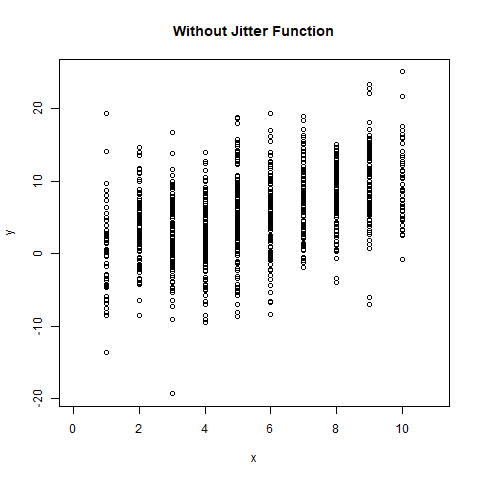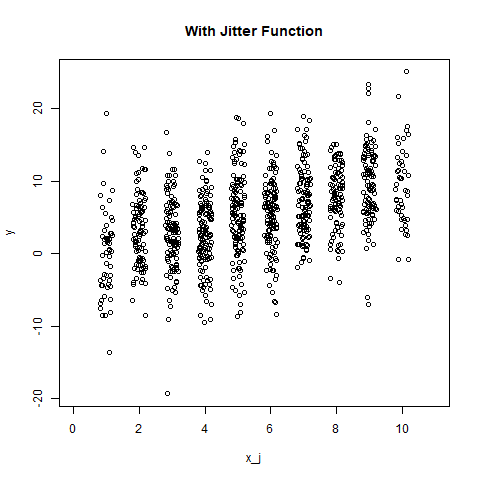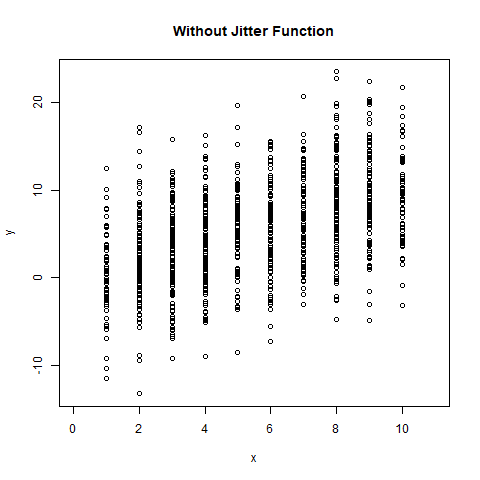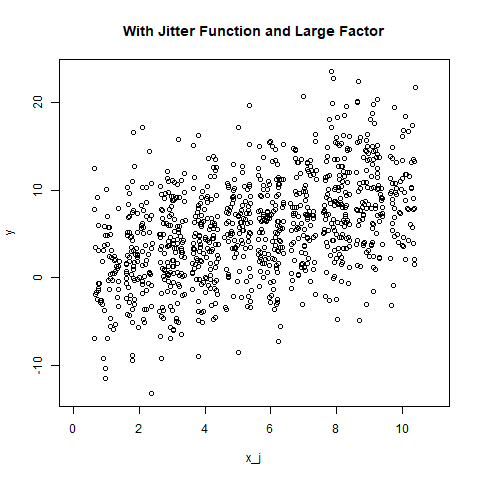# Adding Noise to a Numeric Vector in R Programming – jitter() Function

In R programming, jittering means adding small amount of random noise to a numeric vector object. In this article, we’ll learn to use `jitter()` function and create a plot to visualize them.

Syntax: jitter(x, factor)

Parameters:
x: represents numeric vector
factor: represents numeric value for factor specification

Example 1:

 `# Define numeric vectors ` `x <- ``round``(``runif``(1000, 1, 10)) ` `y <- x + ``rnorm``(1000, mean = 0, sd = 5) ` ` `  `# output to be present as PNG file  ` `png``(file=``"withoutJitter.png"``) ` ` `  `# Plotting without jitter function ` `plot``(x, y, xlim = ``c``(0, 11), ` `     ``main = ``"Without Jitter Function"``) ` ` `  `# saving the file  ` `dev.off``() ` ` `  `x_j <- ``jitter``(x) ` ` `  `# output to be present as PNG file  ` `png``(file=``"withJitter.png"``) ` ` `  `# Plotting with jitter function ` `plot``(x_j, y, xlim = ``c``(0, 11), ` `     ``main = ``"With Jitter Function"``) ` ` `  `# saving the file  ` `dev.off``() `

Output:Example 2: With large factor value

 `# Define numeric vectors ` `x <- ``round``(``runif``(1000, 1, 10)) ` `y <- x + ``rnorm``(1000, mean = 0, sd = 5) ` ` `  `# output to be present as PNG file  ` `png``(file=``"withoutJitterFactor.png"``) ` ` `  `# Plotting without jitter function ` `plot``(x, y, xlim = ``c``(0, 11), ` `     ``main = ``"Without Jitter Function"``) ` ` `  `# saving the file  ` `dev.off``() ` ` `  `x_j <- ``jitter``(x, factor = 2) ` ` `  `# output to be present as PNG file  ` `png``(file=``"withJitterFactor.png"``) ` ` `  `# Plotting with jitter function ` `plot``(x_j, y, xlim = ``c``(0, 11), ` `     ``main = ``"With Jitter Function and Large Factor"``) ` ` `  `# saving the file  ` `dev.off``()`

Output:My Personal Notes arrow_drop_upCheck out this Author's contributed articles.

If you like GeeksforGeeks and would like to contribute, you can also write an article using contribute.geeksforgeeks.org or mail your article to contribute@geeksforgeeks.org. See your article appearing on the GeeksforGeeks main page and help other Geeks.

Please Improve this article if you find anything incorrect by clicking on the "Improve Article" button below.

Article Tags :

Be the First to upvote.

Please write to us at contribute@geeksforgeeks.org to report any issue with the above content.